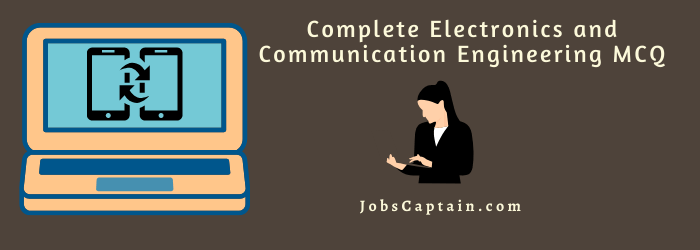# Electronics and Communication Engineering MCQYour welcome to this quick Electronics and Communication Engineering MCQ Quiz. It has 30 basic questions about various Electronics and Communication Engineering topics. Let’s get started.

As you know this Quiz Questions Answer are the most important subject for Electronics and Communication Engineering class students.

Question 1: What is an electronic measuring equipment used in navigation operating in 1GHz band which provides bearing and distance indication?

(A) TACAN

(B) ODR

(C) ILS

(D) DME

(A) TACAN

Question 2:  Find the output of four (4) lead acid cells.

(A) 3.2 V

(B) 8.4 V

(C) 5.8 V

(D) 1.6 V

(B) 8.4 V

Question 3: The reciprocal of capacitance is called ___________ .

(A) elastance

(B) permittivity

(C) conductance

(D) permeability

(A) elastance

Question 4: An advantage of full-wave rectifier over half-wave rectifier.

(A) Each diode can cool-off during half of each input cycle.

(B) The ripple frequency is lower.

(C) The tube will conduct during both halves of the cycle.

(D) Output voltage is lower with more ripples.

(C) The tube will conduct during both halves of the cycle.

Question 5: What composes all matter whether a liquid, solid or gas?

(A) Atoms

(B) Electrons

(C) Protons

(D) Neutrons

(A) Atoms

Question 6: What is the majority carrier in an N material?

(A) Holes

(B) Neutrons

(C) Electrons

(D) Protons

(C) Electrons

Question 7: A very high resistance connected in parallel with smoothing capacitors in a high voltage DC system is called a _________ .

(A) dropping resistor

(B) shunt

(C) divider

(D) bleeder

(D) bleeder

Question 8: What is the smallest part of a computer language?

(A) Binary

(B) Byte

(C) Bit

(D) Word

(C) Bit

Question 9: A user-program that has the ability to move a robot axis to any position within its range ___________ .

(A) Point-to-point

(B) Continuous path

(C) Positive stop

(D) Pick-and-place

(A) Point-to-point

Question 10: What level is used to represent logic-1 in a negative logic circuit?

(A) Negative transition level

(B) Low level

(C) Positive transmission level

(D) High level

(B) Low level

Question 11: What law in electronics where an induced current will be in such a direction that its own magnetic field will oppose the magnetic field that produces the same?

(A) Electromagnetic law

(B) Nortons law

(C) Lenz law

(D) Maxwells law

(C) Lenz law

Question 12: What is the reason why robot actuators have lesser capabilities than electric or hydraulic robot actuators?

(A) It has quick response

(B) It always has oil leaks

(C) It always has all or nothing motion

(D) It has high initial cost

(C) It always has all or nothing motion

Question 13: __________ has the unit of electron volt.

(A) Charge

(B) Potential difference

(C) Energy

(D) Current

(C) Energy

Question 14: What is the unit of magnetic flux in SI system?

(A) Weber

(B) Maxwell

(C) Tesla

(D) Gauss

(A) Weber

Question 15: What element possesses four valence electrons?

(A) Insulator

(B) Semi-insulator

(C) Semiconductor

(D) Conductor

(C) Semiconductor

Question 16: What level is used to represent logic-0 in a negative logic circuit?

(A) High level

(B) Low level

(C) Negative transition level

(D) Positive transition level

(A) High level

Question 17: In an Amplitude-Modulated (AM) radio transmitter, the modulator is ____________ .

(A) How amplification can be thought of as a decision making process

(B) How semiconductor replace vacuum

(C) A digital decision

(D) How to adapt a radio transmitter to make it useful as multiplier circuit

(A) How amplification can be thought of as a decision making process

Question 18: Which of the following is the probable output if all inputs of a TTL gate are binary 1?

(A) Determinable

(B) Binary 0

(C) Binary 1

(D) Indeterminate

(B) Binary 0

Question 19: Term used to describe sudden reverse conduction of an electronic component caused by excess reverse voltage across the device.

(A) Cut-off

(B) Saturation

(C) Avalanche

(D) Revertion

(C) Avalanche

Question 20: Given the two voltages:
S(t)=10 cos(mt+30 degrees)volts
Q(t)=15 cos(mt+45 degrees)volts
Find V(t)=S(t) + Q(t)

(A) V(t)=5.9 cos(mt+71 degrees)volts

(B) V(t)=24.8 cos(+t+39 degrees)volts

(C) V(t)=25 cos(mt+75 degrees)volts

(D) V(t)=13.6 cos(mt+75 degrees)volts

(B) V(t)=24.8cos(+t+39 degrees)volts

Question 21: What type of materials formed when trivalent material is doped with silicon or germanium?

(A) N type

(B) N and P type

(C) PN type

(D) P type

(D) P type

Question 22: What is the degree of exactness of measurement when compared to the expected value of the variable being measured?

(A) Accuracy

(B) Error

(C) Deviation

(D) Precision

(A) Accuracy

Question 23: The overall gain of an amplifier in cascade is ___________ .

(A) the sum

(B) the average of each

(C) the product

(D) 100 % the sum

(C) the product

Question 24: __________ is a byte of data stored in a memory location.

(A) 8 bits

(B) Character

(C) 4 bits

(D) Memory word

(D) Memory word

Question 25: An instrument used to measure one location in terms of coordinates.

(A) Global Positioning System

(B) Hydrometer

(C) Altimeter

(D) Increductometer

(A) Global Positioning System

Question 26: How do you measure the current in a circuit without an ammeter?

(A) By computing the values of resistance

(B) Measure voltage drop across tube

(C) Measure voltage drop across known resistor

(D) Divide total circuit resistance by the total circuit load

(C) Measure voltage drop across known resistor

Question 27: _______ is retrieving data from memory.

(A) Accessing

(B) Getting

(C) Encoding

Question 28: Materials with permeabilities slightly less than that of free space are referred to as __________ .

(A) diamagnetic

(B) ferromagnetic

(C) non-magnetic

(D) paramagnetic

(A) diamagnetic

Question 29: _________ can erase EPROMS.

(A) Applying a 21-volt pulse

(B) Applying ultraviolet rays

(C) Turning off the power

(D) Blowing fuse

(B) Applying ultraviolet rays

Question 30: What materials possess permeabilities slightly less than that of free space?

(A) Non-magnetic

(B) Diamagnetic

(C) Paramagnetic

(D) Ferromagnetic